## MTSO October NewsWe join you in feeling great gratitude this month of October. Helping others that may need a helping hand. Remembering that gratitude opens the heart, heals the body and mind and keeps us in good spirits!

This month is about warmth and healing heat. We have featured products that will help your clients stay warm and heal with heat.

Looking for upgrades to your clinic or home office? Call us at 780-440-1818 for suggestions on how to update your workspace with an affordable electric table, a sweet aromatherapy program, or new sheets.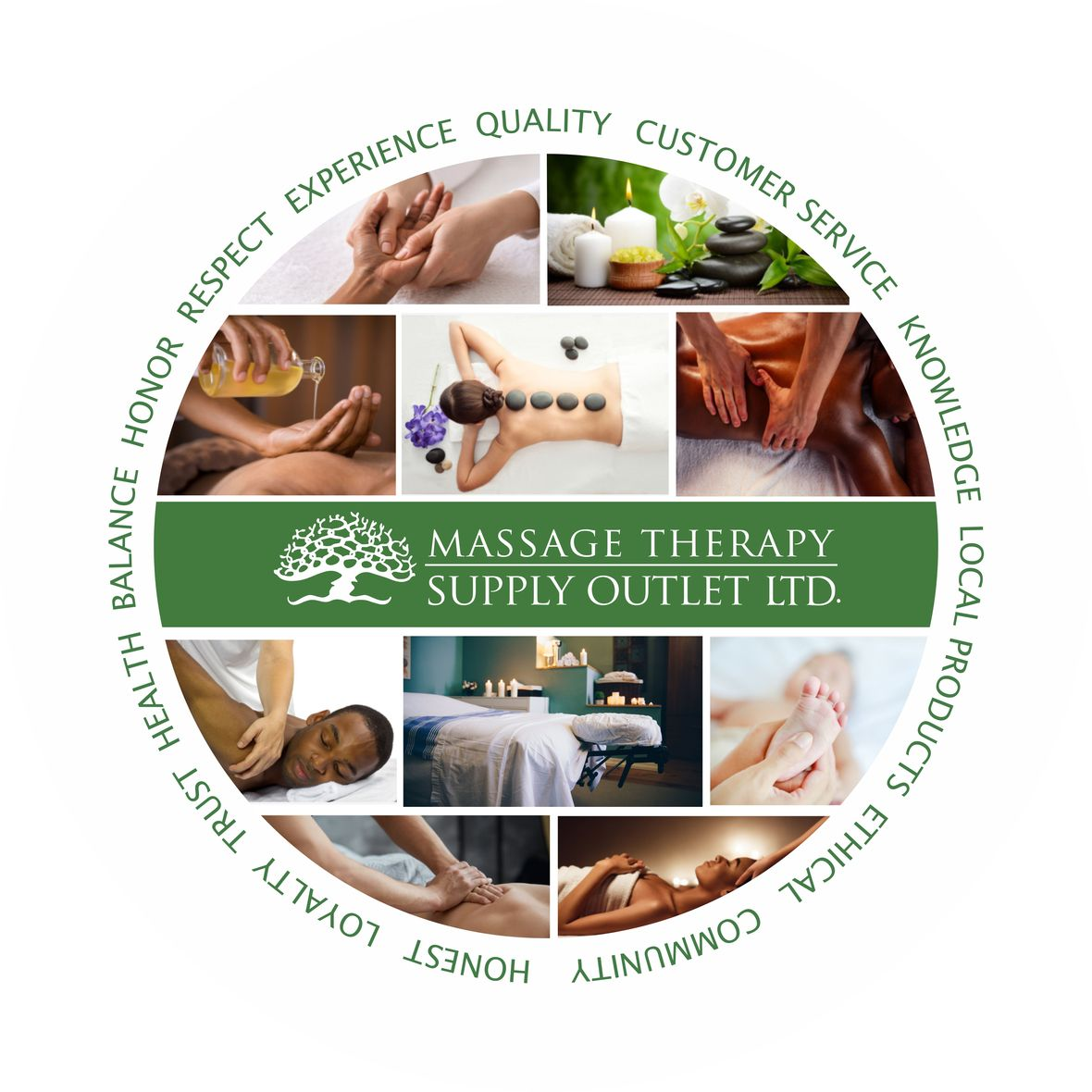table div table+table+table+table+table div table{width:100%;padding:0}table div table+table+table+table+table div table img{width:96.23%;padding:0;float:none}table div table+table+table+table+table div table td{width:100%;padding:0 1.88% 18px}/* styles *//* styles */ MTSO hosted an Outdoor Community Concert on September 19th, featuring the Thom Golub Quartet in our wonderful garden. The audience enjoyed listening to jazz music under the canopy of fall leaves, flowers, warm weather and great company. This event brought community together for the love of music in the great outdoors.table div table+table+table+table+table+table+table+table div table{width:100%;padding:0}table div table+table+table+table+table+table+table+table div table img{width:96.23%;padding:0;float:none}table div table+table+table+table+table+table+table+table div table td{width:100%;padding:0 1.88% 18px}/* styles */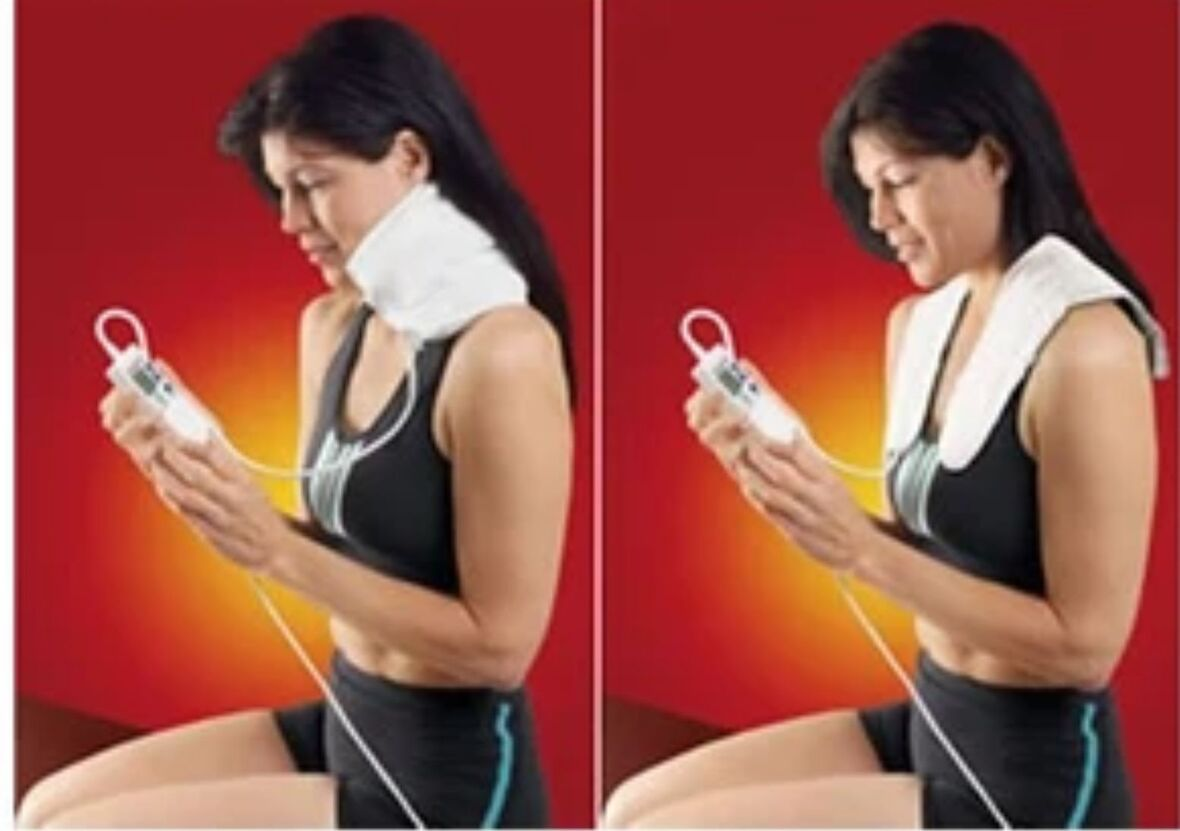THERATHERM DIGITAL MOIST HEAT PAD- SHOULDER

# THERATHERM DIGITAL MOIST HEAT PAD- SHOULDER

\$125.00
Theratherm Digital Moist Heat Pack provides intense, moist heat therapy for temporary relief from pain associated with arthritis, back pain caused by muscle spasm and inflammation caused by strain and tension. Its flannel cover draws moisture from the air and as the pack’s temperature rises, the moisture is released onto the skin, speeding relief to your patients. Its unique, easy-to-use digital control is convenient and safe.

 /* styles */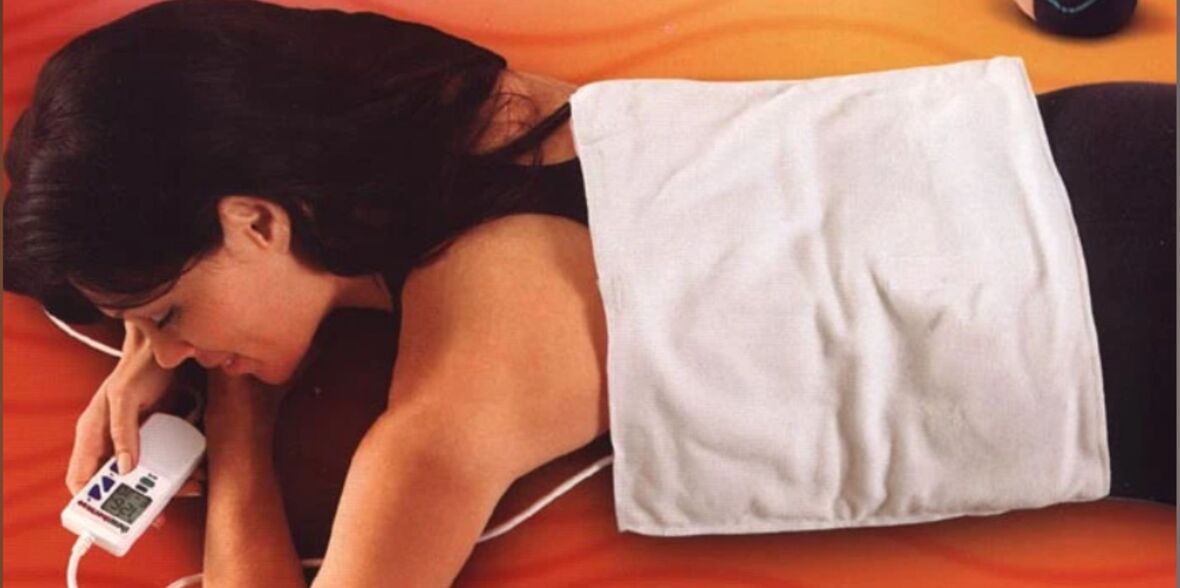THERATHERM DIGITAL MOIST HEAT PACK- LARGE 14" X 27"

# THERATHERM DIGITAL MOIST HEAT PACK- LARGE 14" X 27"

\$129.95
Theratherm Digital Moist Heat Pack - Large that is perfect for your back. Ideal for home use. Keep one at home for yourself, and one in your office for those patients who keep asking, "Where can I buy this?" Works in the same way as the thermaphore.

 table div table+table+table+table+table+table+table+table+table+table+table+table+table+table div table{width:100%;padding:0}table div table+table+table+table+table+table+table+table+table+table+table+table+table+table div table img{width:96.23%;padding:0;float:none}table div table+table+table+table+table+table+table+table+table+table+table+table+table+table div table td{width:100%;padding:0 1.88% 18px}/* styles */# ELECTRIC TABLE WARMER DELUXE

\$130.00

Massage Table Warmer. Provides gentle and comfortable warmth during massages.

Full size (33"x 73") table warmer quickly and evenly distributes the heat.

Ultra Soft and luxurious fleece pad
2 table positioning straps on either end
5 temperature settings
Timer setting 1-10
Auto overheating protection
Thickly padded quilted top gives extra comfort.

 table div table+table+table+table+table+table+table+table+table+table+table+table+table+table+table+table+table div table{width:100%;padding:0}table div table+table+table+table+table+table+table+table+table+table+table+table+table+table+table+table+table div table img{width:96.23%;padding:0;float:none}table div table+table+table+table+table+table+table+table+table+table+table+table+table+table+table+table+table div table td{width:100%;padding:0 1.88% 18px}/* styles */\$140.00
Feel the quality. Feel the comfort. Feel the difference. OAKWORKS Premium Table Warmer

▪ Full Size: 72"x 30" fits most tables
▪ Distributes heat evenly
▪ Programmable temperature
▪ settings from 86° to an incredible 130°
▪ Continuous real time temperature read out
▪ Rapid warming feature
▪ Programmable auto shut-off feature, (up to 99 minutes)
 ▪ Full Size: 72"x 30" fits most tables
 ▪ Distributes heat evenly
 ▪ Programmable temperature
 ▪ settings from 86° to an incredible 130°
 ▪ Continuous real time temperature read out
 ▪ Rapid warming feature
 ▪ Programmable auto shut-off feature, (up to 99 minutes)
 table div table+table+table+table+table+table+table+table+table+table+table+table+table+table+table+table+table+table+table+table div table{width:100%;padding:0}table div table+table+table+table+table+table+table+table+table+table+table+table+table+table+table+table+table+table+table+table div table img{width:96.23%;padding:0;float:none}table div table+table+table+table+table+table+table+table+table+table+table+table+table+table+table+table+table+table+table+table div table td{width:100%;padding:0 1.88% 18px}/* styles */# MASSAGE OIL WARMER

\$55.00
Our premium quality massage oil warmer.
Preferred Features

▪ Replaceable Massage Oil Bottle
▪ Able to Warm Massage Oil, Creme and Lotion
▪ Compact and super lightweight makes it handy and portable
▪ Automatic temperature control
▪ 20 minutes to reach constant temp 60 c/ 140 F
▪ One high quality HDPE massage oil bottle included
 ▪ Replaceable Massage Oil Bottle
 ▪ Able to Warm Massage Oil, Creme and Lotion
 ▪ Compact and super lightweight makes it handy and portable
 ▪ Automatic temperature control
 ▪ 20 minutes to reach constant temp 60 c/ 140 F
 ▪ One high quality HDPE massage oil bottle included
 table div table+table+table+table+table+table+table+table+table+table+table+table+table+table+table+table+table+table+table+table+table+table+table div table{width:100%;padding:0}table div table+table+table+table+table+table+table+table+table+table+table+table+table+table+table+table+table+table+table+table+table+table+table div table img{width:96.23%;padding:0;float:none}table div table+table+table+table+table+table+table+table+table+table+table+table+table+table+table+table+table+table+table+table+table+table+table div table td{width:100%;padding:0 1.88% 18px}/* styles */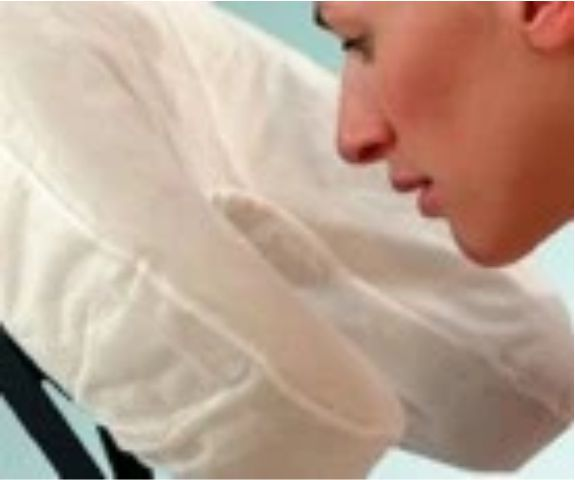Fitted Disposable Face Cradle Covers

# FITTED DISPOSABLE FACE CRADLE COVERS

Made from soft, 25 gsm spunbound fabric, this new and innovative design provides a comfortable, sanitary and disposable barrier for your clients and an easy changeover for between client protection.

▪ Fits all crescent-shaped face rests
▪ Stays put throughout your treatment
▪ Great for table and seated massage
▪ Box of 50
▪ Latex Free

 table div table+table+table+table+table+table+table+table+table+table+table+table+table+table+table+table+table+table+table+table+table+table+table+table+table+table div table{width:100%;padding:0}table div table+table+table+table+table+table+table+table+table+table+table+table+table+table+table+table+table+table+table+table+table+table+table+table+table+table div table img{width:96.23%;padding:0;float:none}table div table+table+table+table+table+table+table+table+table+table+table+table+table+table+table+table+table+table+table+table+table+table+table+table+table+table div table td{width:100%;padding:0 1.88% 18px}/* styles */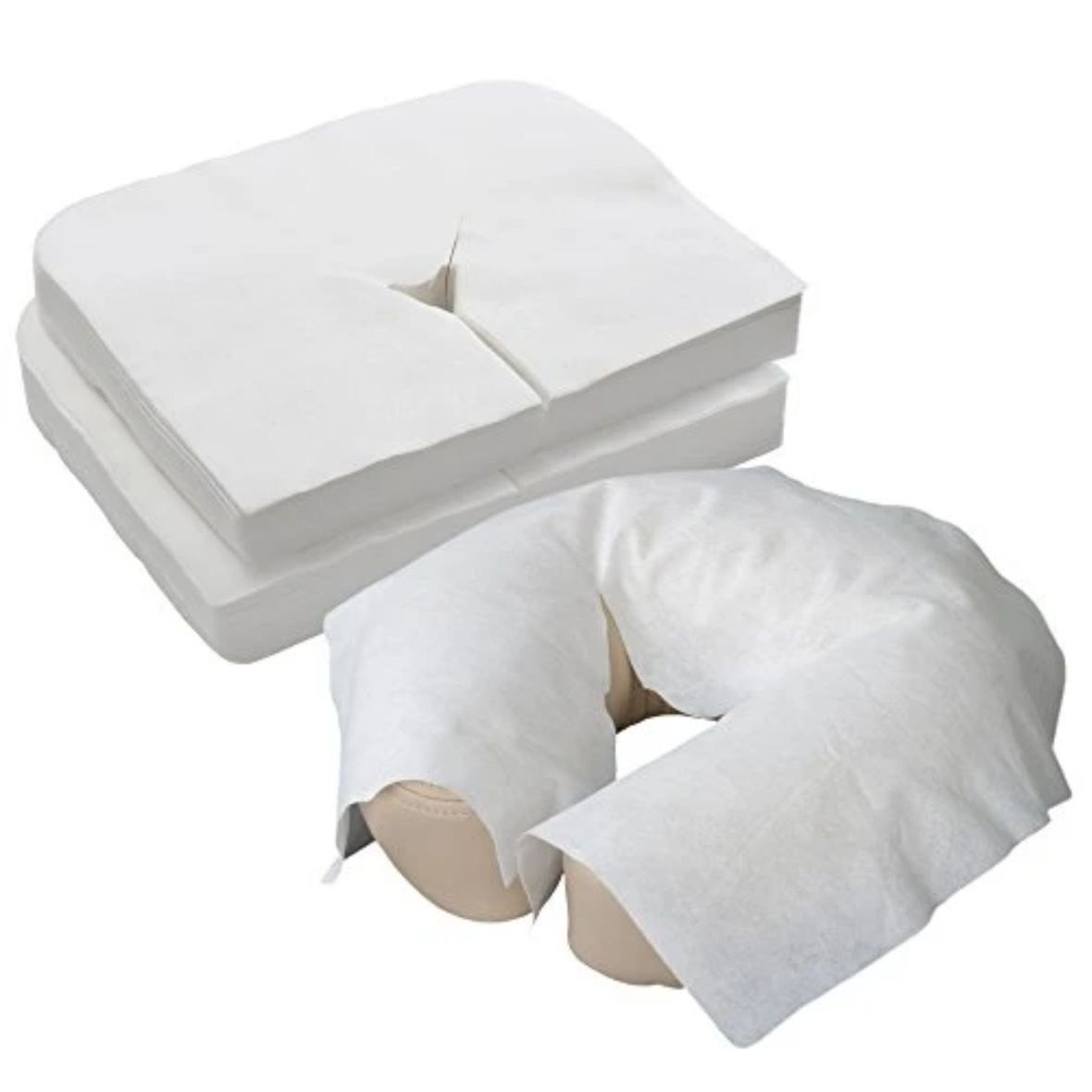Flat Disposable Face Cradle Covers

# FLAT DISPOSABLE FACE CRADLE COVERS

Flat soft disposable face cradle covers package of 100. Product stores easily in table and chair carry cases. Thick, yet soft and silky fabric conforms to the shape of your cushion. Feels like napped cotton. Flat face covers fit almost anything.

 table div table+table+table+table+table+table+table+table+table+table+table+table+table+table+table+table+table+table+table+table+table+table+table+table+table+table+table+table+table div table{width:100%;padding:0}table div table+table+table+table+table+table+table+table+table+table+table+table+table+table+table+table+table+table+table+table+table+table+table+table+table+table+table+table+table div table img{width:96.23%;padding:0;float:none}table div table+table+table+table+table+table+table+table+table+table+table+table+table+table+table+table+table+table+table+table+table+table+table+table+table+table+table+table+table div table td{width:100%;padding:0 1.88% 18px}/* styles */table.module-29{width:95.85%;padding:0}table div table+table+table+table+table+table+table+table+table+table+table+table+table+table+table+table+table+table+table+table+table+table+table+table+table+table+table+table+table+table div table{width:95.85%;float:none;margin-left:auto;margin-right:auto;padding:0}table div table+table+table+table+table+table+table+table+table+table+table+table+table+table+table+table+table+table+table+table+table+table+table+table+table+table+table+table+table+table div table a{border:0 none;text-decoration:none}table div table+table+table+table+table+table+table+table+table+table+table+table+table+table+table+table+table+table+table+table+table+table+table+table+table+table+table+table+table+table div table img{width:100%!important;border:0 none;text-decoration:none}table div table+table+table+table+table+table+table+table+table+table+table+table+table+table+table+table+table+table+table+table+table+table+table+table+table+table+table+table+table+table div table td{width:100%;padding:0}/* styles */
 table div table+table+table+table+table+table+table+table+table+table+table+table+table+table+table+table+table+table+table+table+table+table+table+table+table+table+table+table+table+table+table+table div table{width:100%;padding:0}table div table+table+table+table+table+table+table+table+table+table+table+table+table+table+table+table+table+table+table+table+table+table+table+table+table+table+table+table+table+table+table+table div table img{width:96.23%;padding:0;float:none}table div table+table+table+table+table+table+table+table+table+table+table+table+table+table+table+table+table+table+table+table+table+table+table+table+table+table+table+table+table+table+table+table div table td{width:100%;padding:0 1.88% 18px}/* styles */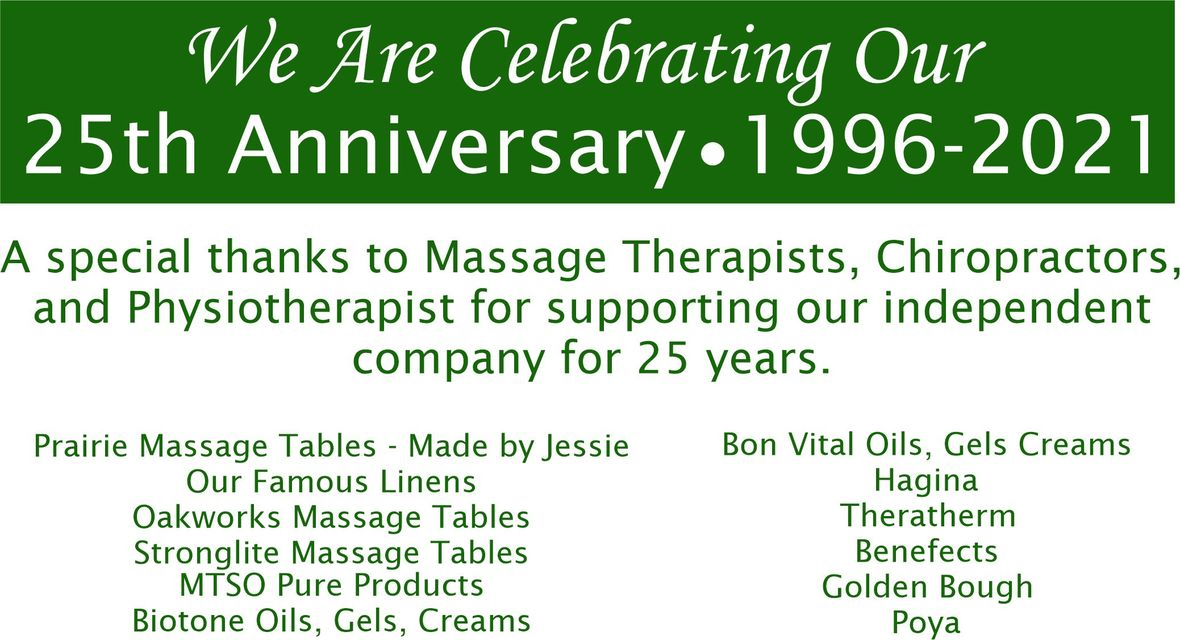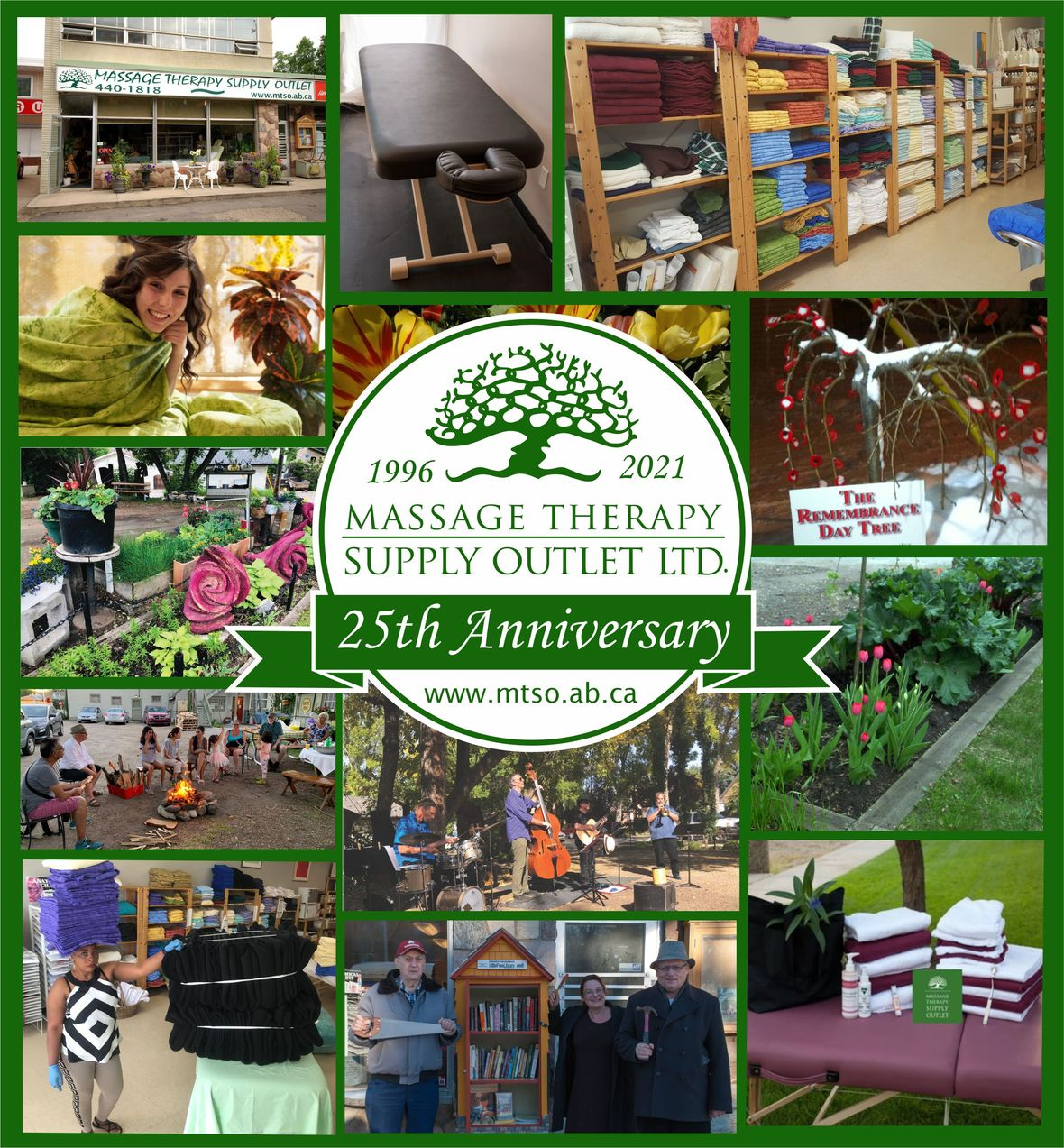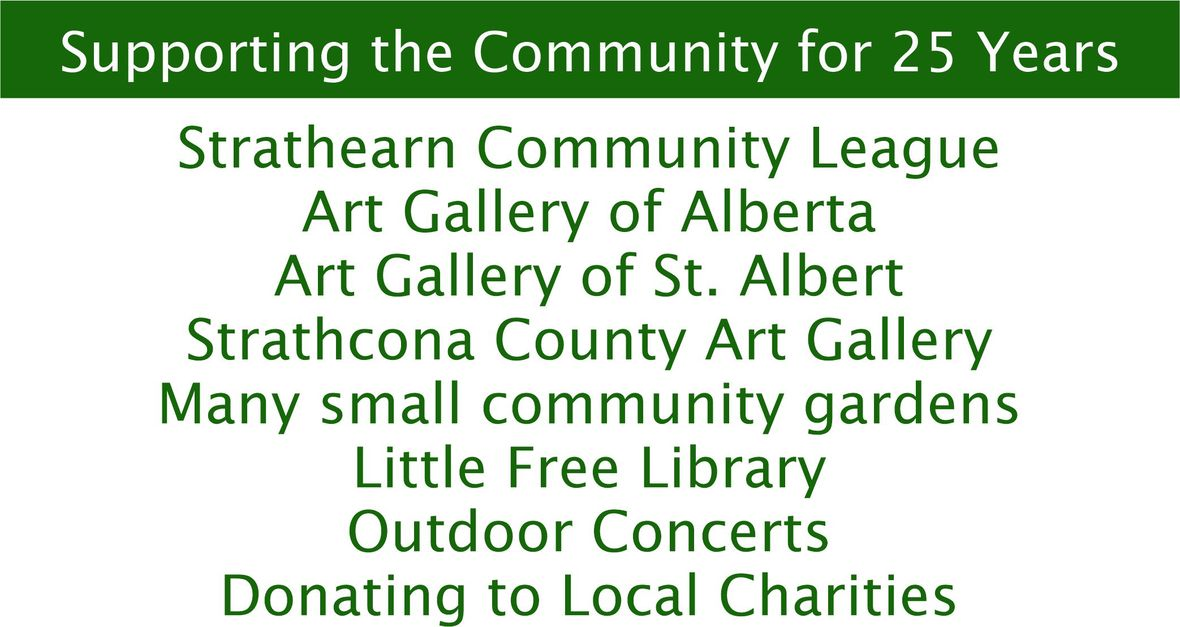table div table+table+table+table+table+table+table+table+table+table+table+table+table+table+table+table+table+table+table+table+table+table+table+table+table+table+table+table+table+table+table+table+table+table+table+table div table{width:100%;padding:0}table div table+table+table+table+table+table+table+table+table+table+table+table+table+table+table+table+table+table+table+table+table+table+table+table+table+table+table+table+table+table+table+table+table+table+table+table div table img{width:96.23%;padding:0;float:none}table div table+table+table+table+table+table+table+table+table+table+table+table+table+table+table+table+table+table+table+table+table+table+table+table+table+table+table+table+table+table+table+table+table+table+table+table div table td{width:100%;padding:0 1.88% 18px}/* styles */# CURBSIDE PICKUP IS AVAILABLE

In addition to in-store shopping and shipping, curbside pick-up is also available. You can either phone-in your order or place order online. When placing your order online choose Local Pick Up at check out. We will contact you when your order is ready.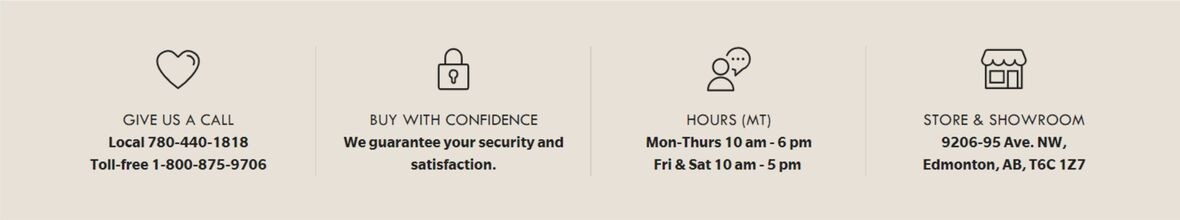/* styles */ Browse and buy from the extensive collection of quality products at our online store, always open, 24/7/365. Drop by our Edmonton store and showroom: : Mon-Fri: 10:00-6:00, Sat: 10:00-5:00
 Like   Tweet   Pin   in